# Fruit equation

Find how many peaches could be bought instead of buying one melon, one pear, one apple and two plums if
we know that:
• Melon and plum cost the same as three apples
• if we subtract the price of a pear from the price of peach, we get the price of plum
• Two peaches and one pear stand together just like a melon and an apple
• Apple and pear stand together just like plum and peach together.

x =  4

### Step-by-step explanation: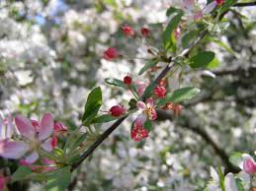Did you find an error or inaccuracy? Feel free to write us. Thank you!Tips to related online calculators
Do you have a system of equations and looking for calculator system of linear equations?

## Related math problems and questions:In six baskets, the seller has fruit. In individual baskets, there are only apples or just pears with the following number of fruits: 5,6,12,14,23 and 29. "If I sell this basket," the salesman thinks, "then I will have just as many apples as a pear." Whic
• Apples and pearsApples cost 50 cents piece, pears 60 cents piece, bananas cheaper than pears. Grandma bought 5 pieces of fruit. There was only one banana, and I paid 2 euros 75 cents. How many apples and how many pears?
• We bought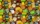We bought 140 fruit, 196 chocolate and 84 caramel lollipops. How many of the same packages can we prepare from them?
• Peaches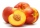There are 20 peaches in the pocket. 3 peaches are rotten. What is the probability that one of the randomly picked two peaches will be just one rotten?
• Phone number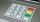Ivan's phone number ends with a four-digit number: When we subtract the first from the fourth digit of this four-digit number, we get the same number as when we subtract the second from the third digit. If we write the four-digit number from the back and
• Apple juice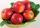From 7 kg of apples we get 3 liters of apple juice. How many liters of juice do we get from 42 kg of apples?
• Sale discountThe product was discounted so that eight products at a new price cost just as five products at an old price. How many percents is the new price lower than the old price?
• Barter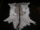There is exchange trade on the market. We know that for two sheepskins we get three goat skins. We also know that for six rabbits skins we get four goat skins. How many rabbit's skins we get for four sheepskins?
• Sequences AP + GPThe three numbers that make up the arithmetic sequence have the sum of 30. If we subtract from the first 5, from the second 4 and keep the third, we get the geometric sequence. Find AP and GP members.
• Miguel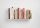Miguel and Kat are reading the same book. Miguel reads three times as many pages of the book as Kat. Together they read 48 pages. Write an equation that can be used to find how many pages Kat read.
• Average weightMom bought 6 apples with an average weight of 130 g - If she took 1 apple out of the bag, the average weight of the remaining apples changed to 140 g. What was the weight of the removed apple?
• Two grandmothersTwo grandmothers went to sell eggs at the market, and they had a total of 100. When they sold all the eggs, they made the same money. The first grandmother says to the second: "If I sold my eggs for your price, I would earn 15 crowns. " The other grandmot
• Shopping vegetablesJohn and his mother were shopping. My mother bought 2 kg of peaches and 5 kg of potatoes and paid CZK 173. The neighbor bought 3 kg of peaches and 4 kg of potatoes and paid CZK 186. How much did 1 kg of peaches and 1 kg of potatoes cost?
• Two-digit numberIn a two-digit number, the number of tens is three greater than the number of units. If we multiply the original number by a number written in the same digits but in reverse order, we get product 3 478. Find the original number.
• Plums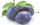By drying 3 kg of fresh plums, we obtained 750 g of dried plums. How many kg of fresh plums need to be dried if we want to get 1.5 kg of dried plums?
• Shoes and slippers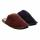Shoes were three times as many as slippers. If shoes were cheaper by 120, they would be twice as expensive as slippers. How much shoes cost and how much slippers?
• PhotosThree friends Mirka, Lucka, and Hanka, collect photos. If Mirka had 3 more photos than she has, she would have as many as Hanka. If Lucka had 5 photos less than she has, she would be like Hanka. How many photographs does each have if they have 431 togethe